3.6.4. Infall Around the Great Attractor: A Case Study

Virialized structures in the Universe produce velocity perturbations of galaxies which are located near them. The amplitude of these perturbations (v / v) depends on the background density of the Universe (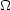) and the density contrast (/) interior to the shell upon which the galaxy is located. The unperturbed velocity in this case is given by v = H0r where r is the distance from the source which is causing the perturbation. The only situation which can be solved analytically (e.g., Regos and Geller 1989, Lightman and Schechter 1990) is the case of a spherical density perturbation in which casev / v can be expanded in powers of/as: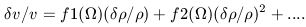(37)

In the linear approximation of Peebles (1980), equation 37 is usually abbreviated to the form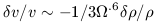(38)

More precise derivations by Regos and Geller (1989) and Lightman and S Schechter (1990) yield values of f1 = -1/3.57 and f2 = 4/63.62. This second order approximation, as well as the fifth order approximation of Regos and Geller, are only valid in the regime of small/. For denser regions it is necessary to employ the exact solution of Regos and Geller (1989). However, Regos and Geller do note that the non-linear approximation of Yahil (1985) tracks the exact solution quite well out to overdensities of20. That approximation is of the form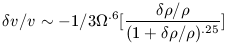(39)

Equation 39 is the standard infall equation that can be used to predict peculiar velocities as a function of radial distance from a virialized structure. To make a prediction a value forhas to be assumed and the radial fall off with distance from the center of the virialized structure of/must be estimated. In general, one assumes that that/falls of as r, where gamma is taken to be 2. We can also define the the turnaround radius (rt) of a virialized structure as the radius at which the inward peculiar velocity of a shell exactly cancels its outward expansion as seen by the center of the virialized structure. This condition requiresv / v = -1.0. In an=1.0 universe, the mean density enclosed within rt is 5.5 (assuming spherical symmetry) , or/= 4.5. Equation 39 predictsv / v = -1 at/= 4.67. The density contrast within rt as a function ofis given by Regos and Geller (1989). In an= 0.1 universe, the density contrast is 29 and hence even the non-linear approximation breaks down before rt is reached. In that sense, infall patterns are easier to treat in the case of= 1.0 as non-linear effects do not quite set in so rapidly.

We now have a framework for analyzing peculiar velocities in terms of a spherical infall model. In principle, such analysis can also determine or constrain. More stringently, the analysis really only constrains.6 / b, where b is the bias parameter used in linear biasing theory. If on the scale of the observations, mass is a perfect tracer of light then b =1 andcan be recovered directly. Studies of peculiar velocities for galaxies in the vicinity of the Hydra-Cen supercluster, however, convincingly show that this procedure is not very straightforward. As such, the history of these studies is an excellent case study of the complex and ambiguous nature of the peculiar velocity field which makes the overall pattern difficult to decipher.

The Hydra-Cen supercluster consists of two main mass concentrations, the Hydra Cluster and the Centaurus cluster. They are denoted by the letters C and H in Figure 3-8 where it is seen that Centaurus is a part of the Super-Galactic plane. The Hydra cluster appears to be unperturbed and is at rest with respect to the CMB with a cosmic velocity of 4055 km s-1. On the other hand, the velocity histogram of Centaurus is strongly bi-modal with one peak occurring at v3000 km s-1 (Cen30) and the other at v4500 km s-1 (Cen45). Using a sample of elliptical galaxies, Faber et al. (1989) report kinematic distances of 2220 ± 250 km s-1 for Cen30 and 3175 ± 335 km s-1 for Cen45. These distances are substantially less than their redshift distances which indicates that Cen30 and Cen45 both have positive peculiar velocities. In conflict with this result are the observations of Lucey and Carter (1988) who measure kinematic distances for a similar sample of elliptical galaxies to determine peculiar velocities of 3115 ± 280 km s-1 for Cen30 and 2675 ± 435 km s-1 for Cen45. These results indicate that Cen30 is at rest and Cen45 is infalling at v2000 km s-1. The opposite case was found from an analysis of the relative distances to spiral galaxies. Using the TF relation, Aaronson et al. (1989) derived mean peculiar velocities of 2835 ± 250 km s-1 for Cen30 and 4260 ± 450 km s-1. In this case, Cen30 exhibits a600 km s-1 velocity residual, while Cen45 would appear to be at rest. Clearly, there is widespread disagreement among the various datasets.

The basis of the GA driven inflow model of Lynden-Bell et al. (1988) is that both Cen 30 and Cen 45 have peculiar velocities with amplitudes of1100 km s-1. If the observed DA of 600 km s-1 for the Milky Way is also the result of GA driven inflow, then we have two values ofv / v at two different distances. With some assumptions, these data can be input to equation 39 to fix the distance of the GA. A GA driven acceleration of the LG with an amplitude of 600 km s-1 requires rt13 Mpc or a mass20 times that of the Virgo cluster. For= 1.0 this corresponds to a mass of 1.3 x 1016 Mwithin r = 13 Mpc. Lynden-Bell et al. (1988) derive a mass of 2.7 x 1016 Mwithin r = 44 Mpc. For= 0.1 the enclosed mass is reduced bya factor of 3. For the smaller scale case of the Virgo cluster, a LG infall velocity of 250 km s-1 requires the Virgo cluster to have a turnaround radius of 4.5-5 Mpc.

As of 1991, there were are two alternatives to the GA driven inflow model which remained consistent with the data. 1) Cen30 is at rest (at v3000 km s-1) and drives an acceleration of the Local Supercluster towards it. This is what was schematically shown in Figure 3-17. 2) Cen30 has the same peculiar velocity (e.g., 600 km s-1) as the Milky Way and is hence participating in the same flow pattern. The simultaneous existence of three very different physical models to the same data set illustrate how ambiguous this problem is. However, a GA driven infall model should be symmetric on both the front and back sides and, as of 1991, only data existed on the front side. The discovery of infall on the backside of the GA would therefore confirm that model.

In 1992 and 1993 the Aaronson et al. group (see Bothun et al. 1992; Mould et al. 1993) published two papers which definitively showed that there was no backside infall towards the hypothesized GA. Other investigators soon reached similar conclusions (e.g., Mathewson et al. 1992). Figure 3-18 summarizes the situation as it presents a comparison of the model infall pattern (equation 39) with the data of Bothun et al. (1992). The GA center distance has been set to be 4350 km s-1, the value proposed by Lynden-Bell et al. (1988). The dashed vertical lines define rt. Points outside of rt are expected to fall-off along the solid lines, which represent the imaginary caustic surface of the virialized structure. The model does not go to 0 at r = 43.5 because we have normalized the model to a value of 600 km s-1 at that distance as we assume the DA is generated by the GA. The dotted lines around the model show the effects of distance errors on the quantityv / v.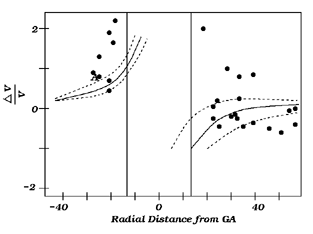Figure 3-18: Individual galaxy peculiar velocities plotted in comparison with the spherically symmetric infall model that would be generated by a Great Attractor located at kinematic distance of 4350 km/s from the Milky Way. The solid curved lines define the front-side and back-side caustic infall surfaces and the dashed lines represent 1-sigma error surfaces. The solid vertical lines represent rt for the Great Attractor. The letter A denotes the Antlia cluster. The model has been normalized such that the Great Attractor accounts for the entire observed dipole anisotropy in the CMB. Figure adapted from Figure 4 in Bothun et al. (1992a).

If infall towards the GA center is responsible for producing the observed amplitude of LG acceleration we would expect the observed data points to fall within the error surfaces surrounding the basic infall model. It is obvious that on the near side the data are generally at much higher peculiar velocity than the model would predict. On the backside, while some points fall within the model predictions many do not and many have the wrong sign for the observed peculiar velocity. To better examine the data set, it is convenient to break it up into three samples which we denote as F (far), N (near), and T (transverse). Sample F is located at a mean distance of 35 Mpc on the backside of the GA center. At this distance, the model predicts Vp = -705 ± 40 km s-1. The data yield Vp = -717 ± 462 km s-1 which is a 1.5detection of backside infall which we do not consider significant. Sample N is located at a mean distance of 21.5 Mpc from the GA on the near side. The model predicts Vp = +1006 ± 30 km s-1 but the data yield Vp = +2366 ± 334 km s-1. Hence, the F and N samples do not indicate any of the required symmetry associated with an infall pattern driven by a virialized structure. Finally, there is the sample T galaxies. These are galaxies whose radial infall to the GA is perpendicular to our line of sight and hence Vp should be zero. However, the measured Vp for this sample is 851 ± 181 km s-1. Thus, the transverse sample does have a significant radial component to its peculiar velocity when, in fact, none should have been observed in the context of symmetric spherical infall towards the GA center.

On balance, the available data seem to rule out the GA model as a viable one that explains the local peculiar velocity field. By itself, the GA model was always worrisome as one could not optically identify a large concentration of light that would correspond to the GA. While the GA is at somewhat low galactic latitude, it sufficiently big in angular extent that obscuration by our own galaxy shouldn't prevent its detection in a redshift survey. At the GA center distance of 4350 km s-1, rt subtends an angle of 16.5°. Figure 3-19 shows the redshift cone diagram in the proposed GA direction for all galaxies with redshifts that are located within the projected turnaround radius of the GA. The very obvious virialized finger belongs to the Centaurus cluster, a structure that was originally measured to have Vp1100 km s-1 and provides the foundation of the GA model. In Figure 3-19 the GA center is located at (0,4350). There is no hint of any virialized structure at this location. Since spherical infall is best generated by virialized structures, the Centaurus cluster is an excellent candidate for contributing to the observed CMB DA. It is hence difficult to understand the observations which suggest that the Centaurus cluster moves in the same 600 km s-1 flow as the LG does.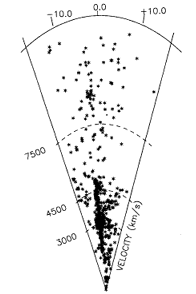Figure 3-19: Cone diagram for all known velocities that are in the putative Great Attractor region of the sky. The large "finger" which is seen is the Centaurus cluster.

To date, the situation with respect to the origin, scale and evolution of peculiar velocities remains confusing. The acquisition of more data by Mould et al. (1993,1995) suggests a picture that is hybrid between that obtained by Aaronson et al. (1986) and the Dn-group. In this scenario, the Hubble flow as delineated by nearby clusters of galaxies is relatively quiet except for samples in the vicinity of large superclusters such as Hydra-Cen or Perseus-Pisces. At these locations, substantial large scale flows are seen with up to 50% of the observed velocity being due to peculiar motions. Figure 3-20 presents a representation of the flow pattern as it has been measured for nearby clusters of galaxies. This flow pattern remains consistent with either bulk flow models or infall driven models with no clear resolution in sight (see also Strauss and Willick 1995).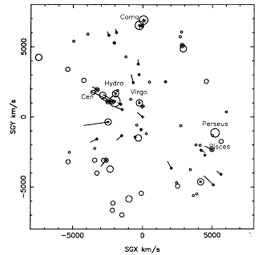Figure 3-20: Distribution of known peculiar velocities for nearby groups and clusters as projected on the plane of the Local Supercluster. Nominal distances are in km/s and the amplitude and direction of any measured peculiar velocity is indicated by the arrows that are tagged onto some groups and clusters. Figure adapted from Mould et al. (1993).